# What information is needed to determine the energy of an electron in a many-electron atom? Check all that apply

What information is needed to determine the energy of an electron in a many-electron atom? Check all that apply.Concepts and reason
The distribution of electron in the atom can be described by quantum numbers. There are 4 types of quantum numbers:

1. Principal quantum number
2. Azimuthal quantum number
3. Magnetic quantum number
4. Spin quantum number

Fundamentals
Principal quantum number: It describes the size of the orbital. It is denoted by the symbol (n). It also describes the energy of the orbital. The value of n = 1, 2, 3….

Azimuthal quantum number: It describes the subparts and shape of the orbital.
It is denoted by the symbol (l). The value of l can be determined from n.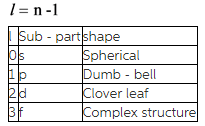Magnetic quantum number: it describes the orientation of orbital in space. It is denoted by the symbol (ml). The range of magnetic quantum number is from –l to +l. l = azimuthal quantum number.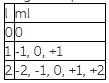Spin quantum number: describes the spin of electrons. It is denoted by the symbol (s). The value of spin quantum number should be either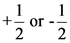.

The energy of an electron in many–electron atom is described by principal quantum number (n) and azimuthal quantum number (l).
The option, ml is wrong because it describes the orientation of orbital in space.
The option, ms is wrong because it describes the spin of electron in orbital.

Information needed to determine the energy of an electron in many–electron atom: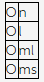The energy of an electron in many–electron atom is determined by both principal quantum number (n) and azimuthal quantum number (l).

The size of an orbital can be described by principal quantum number (n).
The option, l is wrong because it describes the shape of the orbital.
The option, ml is wrong because it describes the orientation of orbital in space.
The option ms is wrong because it describes the spin of electron in orbital.

Information needed to determine the size of an orbital: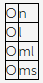The shape of an orbital is determined by principal quantum number (n).

The option, n is wrong because it describes the size and energy of an orbital.
The option, l is wrong because it describes the shape of the orbital.
The orientation of orbital in space is determined by magnetic quantum number (ml).
The option ms is wrong because it describes the spin of electron in orbital.
Information needed to determine the orientation of an orbital: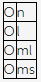The orientation of an orbital is determined by magnetic quantum number (ml).

The option, n is wrong because it describes the size and energy of an orbital.
The shape of the orbital is described by the azimuthal quantum number (l).
The option ml is wrong because it determines the orientation of an orbital in space.
The option ms is wrong because it describes the spin of electron in orbital.

Information needed to determine the general shape of an orbital: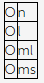The general shape of an orbital is determined by magnetic quantum number (ml).